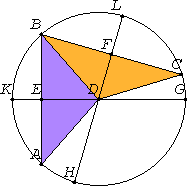# Proposition 9

If a point is taken within a circle, and more than two equal straight lines fall from the point on the circle, then the point taken is the center of the circle.

Let D a point within a circle ABC, and from D let more than two equal straight lines, namely DA and DB and DC, fall on the circle ABC.I say that the point D is the center of the circle ABC.

I.10

Join AB and BC, and bisect them at the points E and F. Join ED and FD, and draw them through to the points G, K, H, and L.

I.8

Then, since AE equals EB, and ED is common, the two sides AE and ED equal the two sides BE and ED, and the base DA equals the base DB, therefore the angle AED equals the angle BED.

Therefore the angles AED and BED are each right. Therefore GK cuts AB into two equal parts and at right angles.

III.1,Cor

And since, if in a circle a straight line cuts a straight line into two equal parts and at right angles, the center of the circle is on the cutting straight line, therefore the center of the circle is on GK.

For the same reason the center of the circle ABC is also on HL.

And the straight lines GK and HL have no other point common but the point D, therefore the point D is the center of the circle ABC.

Therefore if a point is taken within a circle, and more than two equal straight lines fall from the point on the circle, then the point taken is the center of the circle.

Q.E.D.

## Guide

The statement of this proposition is already covered by the last part of Proposition III.7 which says for a point in a circle that is not the center at most two points lie on the circumference at any distance from that point.

This proposition is used in III.25.